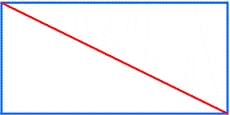# Circumference 7615

The sides of the rectangle are in a ratio of 3: 5. Its circumference is 48 cm. Calculate the length of its diagonal.

u =  17.4929 cm

### Step-by-step explanation:Did you find an error or inaccuracy? Feel free to write us. Thank you!

Tips for related online calculators
Need help calculating sum, simplifying, or multiplying fractions? Try our fraction calculator.
Check out our ratio calculator.
Do you have a linear equation or system of equations and looking for its solution? Or do you have a quadratic equation?
The Pythagorean theorem is the base for the right triangle calculator.